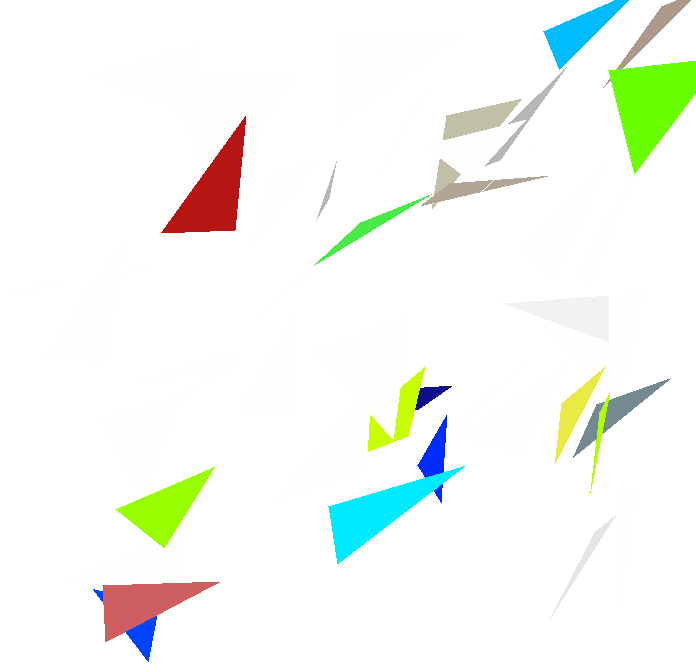# Triangles¶

Generate small triangles randomly in the canvas. Each triangle has a unique id and a random color in the color palette

root > .x triangles.C


Then click on any triangle. A message showing the triangle number and its color will be printed.

Author: Rene Brun
This notebook tutorial was automatically generated with ROOTBOOK-izer from the macro found in the ROOT repository on Sunday, September 19, 2021 at 07:44 AM.

A helper function is created:

In :
%%cpp -d
void TriangleClicked() {
//this action function is called whenever you move the mouse
//it just prints the id of the picked triangle
if (event != 11) return; //may be comment this line
if (!select) return;
if (select->InheritsFrom(TPolyLine::Class())) {
TPolyLine *pl = (TPolyLine*)select;
printf("You have clicked triangle %d, color=%d\n",
pl->GetUniqueID(),pl->GetFillColor());
}
}


Arguments are defined.

In :
Int_t ntriangles=50;

In :
TCanvas *c1 = new TCanvas("c1","triangles",10,10,700,700);
TRandom r;
Double_t dx = 0.2; Double_t dy = 0.2;
Int_t ncolors = gStyle->GetNumberOfColors();
Double_t x,y;
TColor *c;
Int_t ci;
for (Int_t i=0;i<ntriangles;i++) {
x = r.Uniform(.05,.95); y = r.Uniform(.05,.95);
x = x + dx*r.Rndm(); y = y + dy*r.Rndm();
x = x - dx*r.Rndm(); y = y - dy*r.Rndm();
x = x;               y = y;
TPolyLine *pl = new TPolyLine(4,x,y);
pl->SetUniqueID(i);
ci = ncolors*r.Rndm();
c  = gROOT->GetColor(TColor::GetColorPalette(ci));
c->SetAlpha(r.Rndm());
pl->SetFillColor(ci);
pl->Draw("f");
}

gROOT->GetListOfCanvases()->Draw()